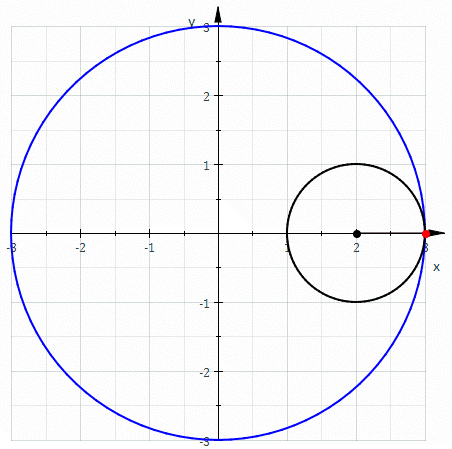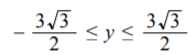# Deltoid Curve

Quartic Curves > Deltoid Curve

A deltoid curve, also called Steiner’s hypocycloid or tricuspoid curve, is a three-cusped Hypocycloid. Hypocycloids are produced by tracing a fixed point on a small circle which rolls within a larger circle; The small circle rolls like a wheel inside the circumference of the bigger circle. The deltoid is produced when the radii of the circles has a ratio of 3:1. In other words, it is produced when a small circle of radius 1 rotates within a circle of radius 3. One other ratio gives a deltoid curve: 3:2.More broadly, a deltoid can be any closed figure with three vertices, connected by curves that are concave to the exterior of the outer circle (which makes the interior points a non-convex set) .

The deltoid curve was first studied by the Swiss mathematicians Leonhard Euler (who discovered the curve while studying optics) and Jakob Steiner, who studied the curve in greater depth; Steiner identified it as the envelope of a family of the Simson lines of a triangle.

It’s thought that it’s called a “deltoid” because it resembled the Greek letter Delta Δ.

## Deltoid Curve Equations and Properties

The parametric equations for the deltoid curve are :

• x = a2cos(θ) + a cos(2θ)
• y = a2sin(θ) – a sin(2θ)
• θ ≤ θ ≤ 2π

Where a is the rolling circle’s radius.

In complex coordinates this becomes z = 2aeit + ae-2it .

Eliminating t from the above equations gives the Cartesian equation:

• (x2 + y2)2 – 8x(x2 – 3y2) + 18(x2 + y2) – 27 = 0.

The length of a deltoid curve is L = 16a and the area is A = 2πa2. The area is exactly half of the unit circle .

The curve has a domain of -3/2 a ≤ x ≤ 3 a and a range ofThe deltoid curve has three singularities, one at each cusp. These can be found at t = 0, ± 2/3.

## Properties: Tangent

The deltoid curve has some interesting properties .Let:

• A = the center of the curve
• B = a cusp
• P = a point on the curve
• E, H = intersection of the curve and tangent at P.
• The line segment EH has constant length [E,H] = 4/3 * [A,B].
• The locus of midpoint D of the tangent segment EH is the inscribed circle.
• E,P,H are concurrent normals; the locus of these intersections is also the inscribed circle.
• If J is the intersection of another tangent that cuts the line segment EH at right angles, the locus traced by J is the inscribed circle.

## References

Deltoid curve illustration: Sam Derbyshire at the English Wikipedia, CC BY-SA 3.0 , via Wikimedia Commons.
 Sexton, E. (1999). The Deltoid Curve. Retrieved January 13, 2021 from: https://mse.redwoods.edu/darnold/math50c/CalcProj/Sp99/Ed/Deltoid2.pdf
 Dayanithi, D. Combination of Cubic and Quartic Plane Curve. IOSR Journal of Mathematics (IOSR-JM)e-ISSN: 2278-5728,p-ISSN: 2319-765X, Volume 6, Issue 2 (Mar. – Apr. 2013), PP 43-53
 Gardner, M. (2020). The Unexpected Hanging and Other Mathematical Diversions
A Classic Collection of Puzzles and Games from Scientific American. American Mathematical Society.
 “Deltoid”. Retrieved January 13, 2021 from: http://virtualmathmuseum.org/docs/Deltoid.pdf

CITE THIS AS:
Stephanie Glen. "Deltoid Curve" From StatisticsHowTo.com: Elementary Statistics for the rest of us! https://www.statisticshowto.com/deltoid-curve/